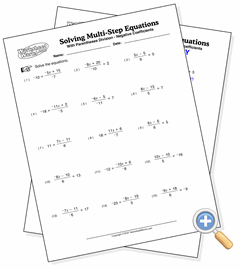# Eliminating Denominators

## Solving Multi-Step EquationsSolve equations involving parentheses

These equations contain values dividing terms with parentheses. The most common solution approach is to multiply both sides of the equation by the value, eliminating the denominator. However they are solved, all problems resolve to integers. You can adjust the difficulty of the problems by increasing the values size, using negative coefficients and flipping the sides of the equations.